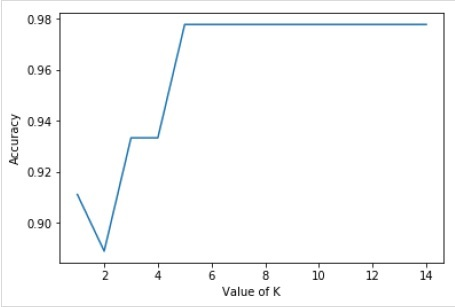# Scikit Learn - KNeighborsClassifier

The K in the name of this classifier represents the k nearest neighbors, where k is an integer value specified by the user. Hence as the name suggests, this classifier implements learning based on the k nearest neighbors. The choice of the value of k is dependent on data. Let’s understand it more with the help if an implementation example −

## Implementation Example

In this example, we will be implementing KNN on data set named Iris Flower data set by using scikit-learn KneighborsClassifer.

• This data set has 50 samples for each different species (setosa, versicolor, virginica) of iris flower i.e. total of 150 samples.

• For each sample, we have 4 features named sepal length, sepal width, petal length, petal width)

First, import the dataset and print the features names as follows −

from sklearn.datasets import load_iris
print(iris.feature_names)


## Output

['sepal length (cm)', 'sepal width (cm)', 'petal length (cm)', 'petal width (cm)']


## Example

Now we can print target i.e the integers representing the different species. Here 0 = setos, 1 = versicolor and 2 = virginica.

print(iris.target)


## Output

[
0 0 0 0 0 0 0 0 0 0 0 0 0 0 0 0 0 0 0 0 0 0 0 0 0 0 0 0 0 0 0 0 0 0 0 0 0
0 0 0 0 0 0 0 0 0 0 0 0 0 1 1 1 1 1 1 1 1 1 1 1 1 1 1 1 1 1 1 1 1 1 1 1 1
1 1 1 1 1 1 1 1 1 1 1 1 1 1 1 1 1 1 1 1 1 1 1 1 1 1 2 2 2 2 2 2 2 2 2 2 2
2 2 2 2 2 2 2 2 2 2 2 2 2 2 2 2 2 2 2 2 2 2 2 2 2 2 2 2 2 2 2 2 2 2 2 2 2
2 2
]


## Example

Following line of code will show the names of the target −

print(iris.target_names)


## Output

['setosa' 'versicolor' 'virginica']


## Example

We can check the number of observations and features with the help of following line of code (iris data set has 150 observations and 4 features)

print(iris.data.shape)


## Output

(150, 4)


Now, we need to split the data into training and testing data. We will be using Sklearn train_test_split function to split the data into the ratio of 70 (training data) and 30 (testing data) −

X = iris.data[:, :4]
y = iris.target
from sklearn.model_selection import train_test_split
X_train, X_test, y_train, y_test = train_test_split(X, y, test_size = 0.30)


Next, we will be doing data scaling with the help of Sklearn preprocessing module as follows −

from sklearn.preprocessing import StandardScaler
scaler = StandardScaler()
scaler.fit(X_train)
X_train = scaler.transform(X_train)
X_test = scaler.transform(X_test)


## Example

Following line of codes will give you the shape of train and test objects −

print(X_train.shape)
print(X_test.shape)


## Output

(105, 4)
(45, 4)


## Example

Following line of codes will give you the shape of new y object −

print(y_train.shape)
print(y_test.shape)


## Output

(105,)
(45,)


Next, import the KneighborsClassifier class from Sklearn as follows −

from sklearn.neighbors import KNeighborsClassifier


To check accuracy, we need to import Metrics model as follows −

from sklearn import metrics
We are going to run it for k = 1 to 15 and will be recording testing accuracy, plotting it, showing confusion matrix and classification report:
Range_k = range(1,15)
scores = {}
scores_list = []
for k in range_k:
classifier = KNeighborsClassifier(n_neighbors=k)
classifier.fit(X_train, y_train)
y_pred = classifier.predict(X_test)
scores[k] = metrics.accuracy_score(y_test,y_pred)
scores_list.append(metrics.accuracy_score(y_test,y_pred))
result = metrics.confusion_matrix(y_test, y_pred)
print("Confusion Matrix:")
print(result)
result1 = metrics.classification_report(y_test, y_pred)
print("Classification Report:",)
print (result1)


## Example

Now, we will be plotting the relationship between the values of K and the corresponding testing accuracy. It will be done using matplotlib library.

%matplotlib inline
import matplotlib.pyplot as plt
plt.plot(k_range,scores_list)
plt.xlabel("Value of K")
plt.ylabel("Accuracy")


## Output

Confusion Matrix:
[
[15 0 0]
[ 0 15 0]
[ 0 1 14]
]
Classification Report:
precision   recall   f1-score    support
0     1.00     1.00        1.00        15
1     0.94     1.00        0.97        15
2     1.00     0.93        0.97        15

micro avg      0.98     0.98        0.98        45
macro avg      0.98     0.98        0.98        45
weighted avg   0.98     0.98        0.98        45

Text(0, 0.5, 'Accuracy')## Example

For the above model, we can choose the optimal value of K (any value between 6 to 14, as the accuracy is highest for this range) as 8 and retrain the model as follows −

classifier = KNeighborsClassifier(n_neighbors = 8)
classifier.fit(X_train, y_train)


## Output

KNeighborsClassifier(
algorithm = 'auto', leaf_size = 30, metric = 'minkowski',
metric_params = None, n_jobs = None, n_neighbors = 8, p = 2,
weights = 'uniform'
)
classes = {0:'setosa',1:'versicolor',2:'virginicia'}
x_new = [[1,1,1,1],[4,3,1.3,0.2]]
y_predict = rnc.predict(x_new)
print(classes[y_predict])
print(classes[y_predict])


## Output

virginicia
virginicia


## Complete working/executable program

from sklearn.datasets import load_iris
print(iris.target_names)
print(iris.data.shape)
X = iris.data[:, :4]
y = iris.target

from sklearn.model_selection import train_test_split
X_train, X_test, y_train, y_test = train_test_split(X, y, test_size=0.30)

from sklearn.preprocessing import StandardScaler
scaler = StandardScaler()
scaler.fit(X_train)
X_train = scaler.transform(X_train)
X_test = scaler.transform(X_test)

print(X_train.shape)
print(X_test.shape)

from sklearn.neighbors import KNeighborsClassifier

from sklearn import metrics

Range_k = range(1,15)
scores = {}
scores_list = []
for k in range_k:
classifier = KNeighborsClassifier(n_neighbors=k)
classifier.fit(X_train, y_train)
y_pred = classifier.predict(X_test)
scores[k] = metrics.accuracy_score(y_test,y_pred)
scores_list.append(metrics.accuracy_score(y_test,y_pred))
result = metrics.confusion_matrix(y_test, y_pred)
print("Confusion Matrix:")
print(result)
result1 = metrics.classification_report(y_test, y_pred)
print("Classification Report:",)
print (result1)
%matplotlib inline
import matplotlib.pyplot as plt
plt.plot(k_range,scores_list)
plt.xlabel("Value of K")
plt.ylabel("Accuracy")

classifier = KNeighborsClassifier(n_neighbors=8)
classifier.fit(X_train, y_train)

classes = {0:'setosa',1:'versicolor',2:'virginicia'}
x_new = [[1,1,1,1],[4,3,1.3,0.2]]
y_predict = rnc.predict(x_new)
print(classes[y_predict])
print(classes[y_predict])